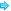科研进展新闻动态科研进展综合新闻传媒扫描
 现在位置：首页 > 新闻动态 > 科研进展

2022-09-09 | 编辑：

Designing privacy-preserving distributed algorithms for stochastic aggregative games is urgent due to the privacy issues caused by information exchange between players. This paper proposes two differentially private distributed algorithms seeking the Nash equilibrium in stochastic aggregative games. By adding time-varying random noises, the input and output-perturbation methods are given to protect each player’s sensitive information. For the case of output-perturbation, utilizing mini-batch methods, the algorithm’s mean square error is inversely proportional to the privacy level ε and the number of samples. For the case of input-perturbation, a differentially private distributed stochastic approximation-type algorithm is developed to achieve almost sure convergence and (ε,δ)-differential privacy. Under suitable consensus time conditions, the algorithm’s convergence rate is rigorously presented for the first time, where the optimal convergence rate O(1/k) in a mean square sense is obtained. Then, utilizing mini-batch methods, the influence of added privacy noise on the algorithm’s performance is reduced, and the convergence rate of the algorithm is improved. Specifically, when the batch sizes and the number of consensus times at each iteration grow at a suitable rate, an exponential rate of convergence can be achieved with the same privacy level. Finally, a simulation example demonstrates the algorithms’ effectiveness.

Publication:

Automatica. Volume 142, August 2022, 110440.

Author:

Jimin Wang

School of Automation and Electrical Engineering, University of Science and Technology Beijing, Beijing, 100083, China

Email: jimwang@ustb.edu.cn

Ji-Feng Zhang

Institute of Systems Science, Academy of Mathematics and Systems Science, Chinese Academy of Sciences, Beijing 100190, China

School of Mathematical Sciences, University of Chinese Academy of Sciences, Beijing 100049, China

Email: jif@iss.ac.cn

Xingkang He

Department of Electrical Engineering, University of Notre Dame, IN 46556, USA

Email: xhe9@nd.edu

【打印本页】【关闭本页】